# 3D-MNIST Image Classification

## Introduction

The MNIST  is the de facto “hello world” dataset for anyone getting started with computer vision. It contains a total of 70,000 examples divided into training and testing sets (6:1). Since its release in 1999, this classic dataset of handwritten images has served as the basis for benchmarking classification algorithms. Various emerging machine learning techniques have been tested with the MNIST, that has remained a reliable resource for researchers and learners alike. More recently, Convolutional Neural Networks (CNN), a class of Deep Neural Networks have demonstrated immense success in the field of computer vision . The MNIST dataset, in particular, has been effectively classified using architectures of this type, with the current state-of-the-art at a high 99.79% accuracy .

If we can get almost perfect accuracy on MNIST, then why study its 3D version?

MNIST is a good database for people who want to get acquainted with computer vision and pattern recognition methods to solve real-world problems, but most of the real-world vision problems like autonomous robots and scene understanding deal with 3D datasets. The availability of 3D data has been increasing rapidly as the use of sensors which provide 3D spatial readings in autonomous vehicles and robots becomes more prevalent. For this reason, the ability of these machines to automatically identify and make inferences about this data has become a crucial task for them to operate autonomously.

Recently, a 3D version of the MNIST was released on Kaggle . The dataset was created by generating 3D point clouds from the images of the digits in the original 2D MNIST. The script for generating point clouds can be found here. This dataset can be considered as a good starting point for getting familiar with 3D vision tasks. In this blog post, we explore this dataset and empirically compare the performance of various machine learning and deep learning based algorithms for the classification of 3D digits.

And most importantly, get familiar with the somewhat less popular 3D-CNN! :)

## Overview of 3D MNIST Dataset

The 3D MNIST dataset is available in HDF5 file format, here. The dataset contains 3D point clouds, i.e., sets of `(x, y, z)` coordinates generated from a portion of the original 2D MNIST dataset (around 5,000 images). The point clouds have zero mean and a maximum dimension range of 1. Each HDF5 group contains:

• points” dataset: `x, y, z` coordinates of each 3D point in the point cloud.

In addition to train and test point clouds, the dataset also contains `full_dataset_vectors.h5` that stores 4096-D vectors obtained from voxelization of all the 3D point clouds and their randomly rotated copies with noise. The `full_dataset_vectors.h5` is splitted into 4 groups:

`>>> X_train = f["X_train"][:]    # shape: (10000, 4096)>>> y_train = f["y_train"][:]    # shape: (10000,)   >>> X_test  = f["X_test"][:]     # shape: (2000, 4096) >>> y_test  = f["y_test"][:]     # shape: (2000,)`

Here is an example to read a digit and store its group content in a tuple.

`>>> with h5.File('train_point_clouds.h5', 'r') as f:      # Reading digit at zeroth index          a = f["0"]         # Storing group contents of digit a      digit = (a["img"][:], a["points"][:], a.attrs["label"])`

Let’s first visualize the contents stored in the tuple with `matplotlib`. The following code plots the first 15 images from the original 2D MNIST with their corresponding labels:

`>>> # Plot some examples from original 2D-MNIST>>> fig, ax = plt.subplots(3,5, figsize=(12, 12), facecolor='w', edgecolor='k')>>> fig.subplots_adjust(hspace = .5, wspace=.2)>>> for ax, d in zip(axs.ravel(), digits):      ax.imshow(d[:])      ax.set_title("Digit: " + str(d))`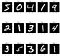First 15 example images along with their labels from the 2D MNIST.

Before visualizing the 3D point clouds, let’s first discuss about Voxelization. Voxelization is the process of conversion of a geometric object from its continuous geometric representation into a set of voxels that best approximates the continuous object. The process mimics the scan-conversion process that rasterizes 2D geometric objects, and is also referred to as 3D scan-conversion.

We use this process to fit an axis-aligned bounding box called the Voxel Grid around the point clouds and subdivide the grid into segments, assigning each point in the point cloud to one of the sub boxes (known as voxels, analogous to pixels). We split the grid into 16 segments along each axis resulting in a total of 4096 voxels, equivalent to the 4th level of an Octree.

`>>> voxel_grid = VoxelGrid(digit, x_y_z = [16, 16, 16])`

The code above generates a voxel grid of `16 × 16 × 16 = 4096` voxels, with the structure attribute representing a 2D array, where each row represents a point in the original point cloud and each column represents the `n_voxel` where it lies with respect to `[x_axis, y_axis, z_axis, global]`.

`>>> voxel_grid.structurearray([ 5, 3, 7, 477])`

The histogram shown below visualize the number of points present within each voxel. From the plot, it can be seen that there are a lot of empty voxels. This is due to the use of a cuboid bounding box to ensure that the Voxel Grid will divide the cloud in a similar way even when the point clouds are oriented to different directions.

`>>> # Get the count of points within each voxel.>>> plt.title("DIGIT: " + str(digits[-1]))>>> plt.xlabel("VOXEL")>>> plt.ylabel("POINTS INSIDE THE VOXEL")>>> count_plot(voxels.structure[:,-1])   # Check definition here.`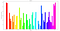Number of Points within each Voxel for a sample of digit 5.

We can visualize the Voxel Grid using the built-in helper function `plot()` defined in the file plot3D.py provided along with the dataset. This function displays the sliced spatial views of the Voxel Grid around the z-axis.

`>>> # Visualizing the Voxel Grid sliced around the z-axis.>>> voxels.plot()>>> plt.show()`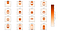Spatial View of the Voxel Grid of a sample of digit 5 sliced around the z-axis.

Now we visualize the 3D point clouds using an open-source 3D software, CloudCompare. We save the Voxel Grid structure as the scalar fields of the original point clouds and render 3D images with CloudCompare.

You can visualize the point clouds with any 3D software of your choice that supports point clouds and scalar fields!

`>>> # Save Voxel Grid Structure as the scalar field of Point Cloud.>>> cloud_vis = np.concatenate((digit, voxel_grid.structure), axis=1)>>> np.savetxt('Cloud Visualization - ' + str(digit) + '.txt', cloud_vis)`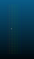Front View, Back View and Side View of 3D rendered image (from point clouds in CloudCompare) for the digit 5.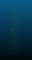Front View, Back View and Side View of 3D rendered image (from point clouds in CloudCompare) for the digit 1.

At last, before moving on to the classification task, just for fun, let’s visualize the animation of 3D data slice by slice. Both the rows of the plot show the animation of slices along `x, y, z-axis` for the digits 5 and 0.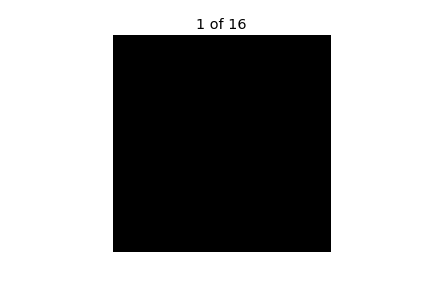Slice by slice animation of 3D voxels around x, y and z-axis for the digits 5 (top) and 3 (bottom).

## Sklearn Machine Learning Classifiers

We use the `full_vectors.h5` dataset. You can use this or generate your own using this script. In machine learning, there’s something called the “No Free Lunch” theorem that in a nutshell, states that no one algorithm works best for every problem. So we try many machine learning algorithms and choose the best suited to our problem.

Let’s start with the simplest yet powerful machine learning classifier — Logistic Regression:

`>>> reg = LogisticRegression()>>> reg.fit(X_train,y_train)>>> print("Accuracy: ", reg.score(X_test,y_test))Accuracy:  0.582`

Logistic Regression provides a decent baseline for other classifiers with an test-accuracy score of 58.2%. Next we move on to Decision Trees:

`>>> dt = DecisionTreeClassifier()>>> dt.fit(X_train,y_train)>>> print("Accuracy: ", dt.score(X_test,y_test))Accuracy:  0.4865`

Decision Trees gives a test-accuracy of 48.65%, i.e. 10 points lower as compared to Logistic Regression. The performance of Decision Trees can possibly be improved with hyper-parameter tuning and grid-search. But here, we stick to the basics and try to get a rough estimate of the performance of the classifier. Moving further, let’s try Support Vector Machines:

`>>> svm = LinearSVC()>>> svm.fit(X_train,y_train)>>> print("Accuracy: ", svm.score(X_test,y_test))Accuracy:  0.557`

We use linear SVMs instead of RBF or Poly kernel based SVM as they take a lot of time to train (you may try training these SVM variants and compare their performance against other classifiers). Linear SVMs perform similar to Logistic Regression with a test-accuracy score of 55.7%. Now, giving K Nearest Neighbors a shot!

`>>> knn = KNN()>>> knn.fit(X_train,y_train)>>> print("Accuracy: ", knn.score(X_test,y_test))Accuracy:  0.5905`

KNN performs best among all the previously tried classifiers with 59.05% test-score. Finally, let’s ensemble; Random Forests:

`>>> rf = RandomForestClassifier(n_estimators=500)>>> rf.fit(X_train,y_train)>>> print("Accuracy: ", rf.score(X_test,y_test))Accuracy:  0.685`

Random Forests outperforms all other classifiers on the classification task. The table below reports the test-accuracy of each classifier on the 3D MNIST dataset. The confusion matrix plots shown below, provides us with the classification report summary of three classifiers namely, Logistic Regression, SVM and Random Forests. From these plots, we observe that most of the classifiers experience difficulty in classifying digits 5, 6 and 9 correctly while find classifying digits like 0, 1 and 4 easy.

You can find the complete code for ML-based empirical analysis here. Although Random Forests performs best among the tried classifiers, the 68.5% mark seems way behind its score on the 2D equivalent dataset. So the natural question that arrives is can we do better?

Can We Do Better? Maybe Yes! 💡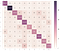Confusion Matrix for Logistic Regression (Left), SVM (Centre), and Random Forests (Right).

## Moving on to Convolutional Neural Networks

Convolutional Neural Networks (CNN), have been immensely successful in classifying the 2D version of MNIST, with the current state-of-the-art model giving a high 99.79% accuracy. So now, let’s see how well these CNN perform with the 3D equivalent of MNIST. We create a 4-layered CNN in keras with two dense layers at the top. For the complete definition of the model, look at the `model()` method. Since a sample in our dataset is a 4096-D voxel and CNN expects a 4D tensor of shape: `(batch_size, height, width, input_channels)`, so we reshape our dataset into a 4D tensor: `(-1, 16, 16, 16)`.

We tried several other shapes such as `(-1, 64, 64, 1)`, `(-1, 64, 64, 3)` but `(-1, 16, 16, 16)` gave the best results, so we stick with this.

`Output ======Epoch 1/303s - loss: 2.0299 - acc: 0.2922 - val_loss: 2.2644 - val_acc: 0.1960Epoch 2/303s - loss: 1.4517 - acc: 0.4913 - val_loss: 2.0336 - val_acc: 0.4440Epoch 3/303s - loss: 1.3081 - acc: 0.5364 - val_loss: 1.6669 - val_acc: 0.5140Epoch 4/303s - loss: 1.2385 - acc: 0.5595 - val_loss: 1.4951 - val_acc: 0.5667Epoch 5/303s - loss: 1.1959 - acc: 0.5799 - val_loss: 1.2812 - val_acc: 0.5813Epoch 6/303s - loss: 1.1682 - acc: 0.5826 - val_loss: 1.1254 - val_acc: 0.6053Epoch 7/303s - loss: 1.1552 - acc: 0.5909 - val_loss: 1.0595 - val_acc: 0.6240Epoch 8/303s - loss: 1.1247 - acc: 0.6008 - val_loss: 0.9993 - val_acc: 0.6460Epoch 9/303s - loss: 1.0981 - acc: 0.6142 - val_loss: 0.9978 - val_acc: 0.6313Epoch 10/303s - loss: 1.0928 - acc: 0.6103 - val_loss: 0.9892 - val_acc: 0.6527Epoch 11/303s - loss: 1.0770 - acc: 0.6202 - val_loss: 0.9940 - val_acc: 0.6387Epoch 12/303s - loss: 1.0627 - acc: 0.6215 - val_loss: 0.9916 - val_acc: 0.6573Epoch 13/303s - loss: 1.0704 - acc: 0.6239 - val_loss: 1.0423 - val_acc: 0.6400Epoch 14/303s - loss: 1.0524 - acc: 0.6296 - val_loss: 0.9560 - val_acc: 0.6573Epoch 15/303s - loss: 1.0331 - acc: 0.6320 - val_loss: 0.9504 - val_acc: 0.6540Epoch 16/303s - loss: 1.0215 - acc: 0.6353 - val_loss: 0.9428 - val_acc: 0.6800Epoch 17/303s - loss: 1.0052 - acc: 0.6461 - val_loss: 0.9492 - val_acc: 0.6633Epoch 18/303s - loss: 1.0090 - acc: 0.6389 - val_loss: 0.9468 - val_acc: 0.6693Epoch 19/303s - loss: 0.9957 - acc: 0.6520 - val_loss: 0.9322 - val_acc: 0.6700Epoch 20/30Epoch 00019: reducing learning rate to 0.000500000023749.3s - loss: 0.9831 - acc: 0.6507 - val_loss: 0.9482 - val_acc: 0.6653Epoch 21/303s - loss: 0.9643 - acc: 0.6636 - val_loss: 0.8976 - val_acc: 0.6767Epoch 22/303s - loss: 0.9341 - acc: 0.6679 - val_loss: 0.8920 - val_acc: 0.6873Epoch 23/303s - loss: 0.9078 - acc: 0.6785 - val_loss: 0.8755 - val_acc: 0.6933Epoch 24/303s - loss: 0.9386 - acc: 0.6650 - val_loss: 0.8968 - val_acc: 0.6840Epoch 25/303s - loss: 0.9130 - acc: 0.6792 - val_loss: 0.8872 - val_acc: 0.6900Epoch 26/303s - loss: 0.8946 - acc: 0.6765 - val_loss: 0.8736 - val_acc: 0.6960Epoch 27/303s - loss: 0.8997 - acc: 0.6785 - val_loss: 0.8668 - val_acc: 0.7060Epoch 28/303s - loss: 0.8802 - acc: 0.6879 - val_loss: 0.8859 - val_acc: 0.6853Epoch 29/303s - loss: 0.8877 - acc: 0.6890 - val_loss: 0.8732 - val_acc: 0.6967Epoch 30/303s - loss: 0.8832 - acc: 0.6858 - val_loss: 0.8768 - val_acc: 0.6913Accuracy : 0.698Model Saved.`

## 3D Convolutional Networks in Keras

Finally, we move onto the main objective of this post (:P), 3D CNN. The 3D CNN model is similar to our 2D CNN model. For the complete definition of the model, check the `model()` method. Now, like with 2D CNN, the 3D CNN expects a 5D tensor of shape `(batch_size, conv_dim1, conv_dim2, conv_dim3, input_channels)`. We reshape out input to a 5D tensor — `(-1, 16, 16, 16, 3)`and feed it to our 3D CNN.

`Output======Epoch 1/3011s- loss: 2.1194 - acc: 0.2912 - val_loss: 2.1434 - val_acc: 0.1660Epoch 2/3010s- loss: 1.3554 - acc: 0.5274 - val_loss: 1.8831 - val_acc: 0.4933Epoch 3/3010s- loss: 1.1520 - acc: 0.5954 - val_loss: 2.0278 - val_acc: 0.3427Epoch 4/3010s- loss: 1.0604 - acc: 0.6272 - val_loss: 1.3859 - val_acc: 0.5500Epoch 5/3010s- loss: 0.9991 - acc: 0.6448 - val_loss: 1.2405 - val_acc: 0.6000Epoch 6/3010s- loss: 0.9305 - acc: 0.6756 - val_loss: 1.0759 - val_acc: 0.6113Epoch 7/3010s- loss: 0.8790 - acc: 0.6939 - val_loss: 1.0644 - val_acc: 0.6673Epoch 8/3010s- loss: 0.8295 - acc: 0.7087 - val_loss: 0.8908 - val_acc: 0.6920Epoch 9/3010s- loss: 0.7648 - acc: 0.7289 - val_loss: 0.9673 - val_acc: 0.6940Epoch 10/3010s- loss: 0.7001 - acc: 0.7542 - val_loss: 0.8410 - val_acc: 0.7047Epoch 11/3010s- loss: 0.6485 - acc: 0.7719 - val_loss: 0.8781 - val_acc: 0.7133Epoch 12/3010s- loss: 0.5999 - acc: 0.7912 - val_loss: 0.8172 - val_acc: 0.7180Epoch 13/3010s- loss: 0.5375 - acc: 0.8147 - val_loss: 0.8366 - val_acc: 0.7207Epoch 14/3010s- loss: 0.4936 - acc: 0.8284 - val_loss: 0.8288 - val_acc: 0.7193Epoch 15/3010s- loss: 0.4337 - acc: 0.8494 - val_loss: 0.8528 - val_acc: 0.7320Epoch 16/3010s- loss: 0.4099 - acc: 0.8593 - val_loss: 1.0491 - val_acc: 0.6907Epoch 17/3010s- loss: 0.3601 - acc: 0.8764 - val_loss: 0.9005 - val_acc: 0.7333Epoch 18/3010s- loss: 0.3492 - acc: 0.8838 - val_loss: 0.9155 - val_acc: 0.7060Epoch 19/3010s- loss: 0.2939 - acc: 0.9000 - val_loss: 1.2862 - val_acc: 0.7007Epoch 20/3010s- loss: 0.2837 - acc: 0.9058 - val_loss: 0.9119 - val_acc: 0.7093Epoch 21/30Epoch 00020: reducing learning rate to 0.000500000023749.10s- loss: 0.2786 - acc: 0.9104 - val_loss: 0.9305 - val_acc: 0.7287Epoch 22/3010s- loss: 0.1521 - acc: 0.9508 - val_loss: 1.0142 - val_acc: 0.7473Epoch 23/3010s- loss: 0.0944 - acc: 0.9699 - val_loss: 1.0704 - val_acc: 0.7300Epoch 24/3010s- loss: 0.0831 - acc: 0.9746 - val_loss: 1.1331 - val_acc: 0.7407Epoch 25/3010s- loss: 0.0691 - acc: 0.9773 - val_loss: 1.1615 - val_acc: 0.7393Epoch 26/30Epoch 00025: reducing learning rate to 0.000250000011874.10s- loss: 0.0665 - acc: 0.9785 - val_loss: 1.1331 - val_acc: 0.7340Epoch 27/3010s- loss: 0.0468 - acc: 0.9856 - val_loss: 1.1230 - val_acc: 0.7367Epoch 28/3010s- loss: 0.0347 - acc: 0.9888 - val_loss: 1.1312 - val_acc: 0.7460Epoch 29/30Epoch 00028: reducing learning rate to 0.000125000005937.10s- loss: 0.0283 - acc: 0.9919 - val_loss: 1.1361 - val_acc: 0.7340Epoch 30/3010s- loss: 0.0285 - acc: 0.9906 - val_loss: 1.1466 - val_acc: 0.7327Accuracy: 0.771Model Saved.`

The performance of 2D CNN is close to Random Forests with a test-score of 69.8%, but 3D CNN outperforms all other classifiers by a significant margin, giving a high 77.1% test-accuracy. Below we summarise the classification reports of 2D CNN and 3D CNN through their confusion matrix plots and learning curves respectively.

## More 3D Datasets

In this blog post, we experimented with 3D MNIST dataset, that proved to be a good benchmark for starting with 3D Datasets. Here is a list of few more 3D Datasets that are worth playing with:

1. Data Science Bowl 2017, available here.

## References

1. The MNIST database of handwritten digits. Y LeCun, C Cortes. 1998.

Written by

## Shashwat Aggarwal

#### Musings on AI, Machine Learning and Deep learning.

Welcome to a place where words matter. On Medium, smart voices and original ideas take center stage - with no ads in sight. Watch
Follow all the topics you care about, and we’ll deliver the best stories for you to your homepage and inbox. Explore
Get unlimited access to the best stories on Medium — and support writers while you’re at it. Just \$5/month. Upgrade